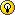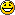Page 4 of 7

### Re: User Functions Exchange

Posted: 02 Jul 2015 01:47
(But the eval was necessary because feeding a decimal number with a dot or a comma made a difference)
True.

Btw, you can even make a new function out of it:

Code: Select all

``function nthroot(\$num, \$n=2) { return eval(\$num) ^ (1/\$n); }``
While \$n must be a natural number (and it should be >= 2)

### Custom range function

Posted: 02 Jul 2015 21:01
Just wrote a quick range function which will allow you to search through number ranges either positively or negatively by custom steps and put into a list. I put check in to protect against people passing with 0 steps (infinite).

Here's the function:

Code: Select all

``````    function range(\$from, \$to, \$step=1, \$seperator="|") {
if (\$step == 0) {
end 1==1, "Value for step [ 0 ] is not an allowed step for range function";
}

\$count = \$from;
\$list = "";
if (\$step > 0) {
while(\$count <= \$to) {
\$list = \$list . "|" . \$count;
\$count = \$count + \$step;
}
} else {
while(\$count >= \$to) {
\$list = \$list . "|" . \$count;
\$count = \$count + \$step;
}
}

return formatlist(\$list, "e");
}
``````
here's how you can use it:

Code: Select all

``````                text range(1, 10) //Will create a text box with 1|2|3|4|5|6|7|8|9|10 in it.

//This will loop through the range and echo 1 then 3 then 5
foreach(\$number, range(1, 5, 2)) {
echo \$number;
}

//This will loop through the range in reverse order and echo 10, 8, 6
foreach(\$number, range(10, 5, -2)) {
echo \$number;
}
``````
[moved]

### Re: User Functions Exchange

Posted: 03 Jul 2015 07:49
Would some mod please make this thread sticky. Thx!

### Re: User Functions Exchange

Posted: 03 Jul 2015 08:21
Only Don can do this...

### Re: User Functions Exchange

Posted: 03 Jul 2015 15:01
Hmm, I could move and rewrite the first two posts of this topic into a new topic "User-defined Functions Collection" for Don to make sticky. In fact I'd already tried that, but discovered I don't have glue.### Re: User Functions Exchange

Posted: 19 Jul 2015 20:30
sendkeyescape(): Escapes a string for use in sendkeys.
So that a % becomes a % and not an Alt key, a {Home} becomes the string {Home} and not the Home key, and so on.

Code: Select all

``````FUNCTION sendkeyescape(\$str) {
/* Escape special sendkeys control characters in a string,
** to enable snedkey to type out the string as-is.
**  \$str   the string to Escape */
\$str = regexreplace(\$str,'([\{\}\^\~\%\+])','{\$1}');
return \$str;
}``````

### Re: User Functions Exchange

Posted: 19 Jul 2015 21:23
Sendkeys - that's my cue, this might be a keeper, gluey stuffNot sure, though, how to benefit from it. What would the following look like when using your function?

Code: Select all

``"Config Backup ..." ::sendkeys "^k{home}fff%n";``

### Re: User Functions Exchange

Posted: 20 Jul 2015 07:50
that will type "^k{home}fff%n" exactly.

### Re: User Functions Exchange

Posted: 20 Jul 2015 07:57got it, string and escape being the keywords here, just like it says### Re: User Functions Exchange

Posted: 21 Jul 2015 10:08
pad() : reciprocally pads two strings (or numbers) to the same length.
In other words, takes two strings, and pads the shorter one up to the same length as the other.
Note that this touches the input variables (passed by reference).

Code: Select all

``````FUNCTION pad(&\$stringA, &\$stringB, \$padding, \$side = 'L', \$trim = 0){
/* reciprocally pad two strings to the same length
**  \$stringA, \$stringA   the two strings
**  \$padding   the padding char, must be one character
**             if \$stringA looks like a number, defaults to 0
**             if \$stringA looks like a string, defaults to ' '
**  \$side      which side to pad, 'L'eft or 'R'ight
**  \$trim      if 1, then ' ' or 0 are trimmed (NOT the padding char)
*/
\$padding = substr(\$padding, 0, 1); //take single padding char
if (\$padding == '') { if (regexmatches(\$stringA, '[^0-9\-\+\.]') == '') {\$padding = 0; } else { \$padding = ' '; } }
if (\$trim == 1) {\$stringA = trim(\$stringA, '0 '); \$stringB = trim(\$stringB, '0 ');}

\$lenA = strlen(\$stringA); \$lenB = strlen(\$stringB); \$diffA = 0; \$diffB = 0; \$diff = (\$lenA - \$lenB);
if (\$diff < 0) {\$diffA = abs(\$diff);} elseif (\$diff > 0) {\$diffB = abs(\$diff);}
\$stringA = (\$diffA == 0) ? (\$stringA) : (((\$side=='L')?strrepeat(\$padding, \$diffA):'') . \$stringA . ((\$side=='R')?strrepeat(\$padding, \$diffA):''));
\$stringB = (\$diffB == 0) ? (\$stringB) : (((\$side=='L')?strrepeat(\$padding, \$diffB):'') . \$stringB . ((\$side=='R')?strrepeat(\$padding, \$diffB):''));
}``````
Examples
assuming the function is saved in <xyscripts>\inc\pad.xyi

Code: Select all

`````` \$a = 'right'; \$b = 'aligned';
pad(\$a, \$b);
text \$a.<crlf>.\$b;
INCLUDE "inc\pad.xyi";
/*returns
right
aligned
*/``````

### Re: User Functions Exchange

Posted: 18 Feb 2016 21:27
these are three related functions

Code: Select all

`````` FUNCTION RemTokenPos(\$string, \$index, \$sep){
// function to remove single token
\$len = gettoken(\$string, 'count', \$sep);
if (\$index <= 0) || (\$index > \$len) { return \$string;}
\$half1=gettoken( \$string, \$index-1, \$sep, 1, 1);
\$half2=gettoken(\$string, \$index+1, \$sep, 1, 2);
if (\$half1=="") { \$string=\$half2;}
elseif (\$half2=="") { \$string=\$half1;}
else {\$string="\$half1\$sep\$half2";}
return \$string;}

FUNCTION RepTokenPos(\$string, \$token, \$index, \$sep){
// function to replace token
\$len = gettoken(\$string, 'count', \$sep);
if (\$index <= 0) || (\$index > \$len) { return \$string;}
\$half1=gettoken( \$string, \$index-1, \$sep, 1, 1);
\$half2=gettoken(\$string, \$index+1, \$sep, 1, 2);
if (\$half1=="") { \$string="\$token\$sep\$half2";}
elseif (\$half2=="") { \$string="\$half1\$sep\$token";}
else {\$string="\$half1\$sep\$token\$sep\$half2";}
return \$string;}

FUNCTION InsTokenPos(\$string, \$token, \$index, \$sep){
// function to insert token
// to insert @ beginning use 0
// else it will insert after the index
// i.e.  \$index=1 will insert @ 2
\$len = gettoken(\$string, 'count', \$sep);
if (\$index < 0) || (\$index > \$len ) { return \$string;}
\$half1=gettoken( \$string, \$index, \$sep, 1, 1);
\$half2=gettoken(\$string, \$index+1, \$sep, 1, 2);
if (\$half1=="") { \$string="\$token\$sep\$half2";}
elseif (\$half2=="") { \$string="\$half1\$sep\$token";}
else {\$string="\$half1\$sep\$token\$sep\$half2";}
return \$string;}``````

Code: Select all

``````"examples "
\$a="19|20|22|22|21";
\$b=5;
\$nToken=30;
\$sep="|";
\$c=RemTokenPos(\$a, \$b, \$sep);
text \$c; //19|20|22|22
\$d=RepTokenPos(\$a, \$nToken, \$b, \$sep);
text \$d; //19|20|22|22|30
\$d=InsTokenPos(\$a, \$nToken, \$b, \$sep);
text \$d; //19|20|22|22|21|30``````
have not fully tested but did see some shocking resultsEdit
Thank you highend. what a basic mistake, should have tested properly

Edit
Thanks to Sammay and PeterH

### Re: User Functions Exchange

Posted: 18 Feb 2016 23:07
All the three functions allow the usage of a separator but you'll return a hardcoded format ("|"- separated).
Either allow two separators (one for the \$string and one for the \$token) or at least rewrite it
to return the format of the source string (and not a hardcoded "|")...

### Re: User Functions Exchange

Posted: 19 Feb 2016 10:40
highend wrote:All the three functions allow the usage of a separator but you'll return a hardcoded format ("|"- separated).
Either allow two separators (one for the \$string and one for the \$token) or at least rewrite it
to return the format of the source string (and not a hardcoded "|")...
Thanks again highend . It certainly got me thinking . First off realized that these functions didn't do the work I intended
to use them for. Secondly why would anyone need a different separator for the \$token.
This is where it gets interesting ,what if you inserted a \$token="(a,b,c)" into \$a .
A BIG THANKS again not just for this but also for all your work/help.

### Re: User Functions Exchange

Posted: 19 Feb 2016 11:00
#1 - Concatenate variables with a '.' to avoid syntax warnings (Scripting > Syntax Checking).

Code: Select all

``\$half1.\$sep.\$token.\$sep.\$half2``
#2 - RemveTokenPos -> RemTokenPos sounds better and consistent with the other two functions, no?

#3 - And perhaps consider adding a check like this to be consistent with builtin gettoken.

Code: Select all

``````//RemTokenPos, RepTokenPos
\$len = gettoken(\$string, 'count', \$sep);
if (\$index <= 0) || (\$index > \$len) { return \$string;}
//InsTokenPos
\$len = gettoken(\$string, 'count', \$sep);
if (\$index < 0) || (\$index > \$len - 1) { return \$string;}``````

### Re: User Functions Exchange

Posted: 19 Feb 2016 11:45
SammaySarkar wrote:#1 - Concatenate variables with a '.' to avoid syntax warnings (Scripting > Syntax Checking).

Code: Select all

``\$half1.\$sep.\$token.\$sep.\$half2``
Very right!

And in this very situation also

Code: Select all

``"\$half1\$sep\$token\$sep\$half2"``
would be correct.
This would even allow you to add some constant characters to the resulting string without changing between quoted and non-quoted notation.
(But beware: in this case any character directly following a variable name must be non-alphabetic, i.e. not be allowed as part of a variable name, so that it isn't taken as part of that name. Further chars can be anything.

Code: Select all

``"Prefix\$half1\$sep\$token\$sep\$half2-Suffix"``
as an example. Note the "-" in front of Suffix - it terminates the variable name "\$half2"!)

So both notations can make sense.